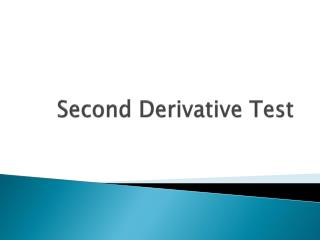DownloadDownload PresentationSecond Derivative Test

# Second Derivative Test

Download Presentation## Second Derivative Test

- - - - - - - - - - - - - - - - - - - - - - - - - - - E N D - - - - - - - - - - - - - - - - - - - - - - - - - - -
##### Presentation Transcript

1. Second Derivative Test

2. Concavity If the graph of a function flies above all of its tangents, then it is called concave upward If the graph of a function flies below all of its tangents, then it is called concave downward

3. Test for Concavity If f’’ > 0 for all x in an interval, then the graph is concave upward If f’’ < 0 for all x in an interval, then the graph is concave downward

4. Example Determine areas of concavity for

5. Example Determine where the graph is concave up and concave down

6. Inflection Points Where a curve changes concavity

7. Example Determine Inflection Points for

8. Second Derivative Test The second derivative can be used to identify local max and min values as well If f’ = 0 and the second derivative exists on an interval 1) If f’’(c)> 0, then this c is a minimum 2) If f’’(c) < 0, then this c is a maximum

9. Example Use the second derivative test to locate the extrema of